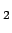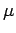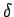: 2 Atmospheric model : Two dimensional anelastic model : $BL\ # 1 Outline of the model The 2D model system consists of the atmosphere and the grand surface. The model atmosphere is composed of ideal gas. The atmospheric constituent is assumed to COonly and its condensation and sublimation are not considered. The values of soil density, thermal conductivity and specific heat are constant each other. The surface topography is not considered. Atmospheric model The governing equation of model atmospheric dynamics is the 2D anelastic system (Ogura and Phillips, 1962). Turbulent parametarization The subgrid turbulent mixingis evaluated by the formula of Klemp and Wilhelmson (1978). The value of turbulent diffusion coefficient for heat is equal to that for momentum. Surface flux The surface momentum and heat fluxes are given by the bulk formulae, where the bulk coefficients depend on static stability and vertical wind shear ( Louis, 1979). The bulk coefficient for heat transport has same value of that for momentum. The roughness length for the bulk coefficients is 1 cm ( Sutton et al., 1978). Dust transport The spatial distribution of dust is calculated by advection diffusion equation which considers gravitational settling of dust. The representation of dust terminal velocity follows Conrath (1975). The radius of dust particle is constant value (0.4m). The value of dust flux from the surface is constant. Radiation The radiative transfer of COatmosphere is calculated by a Goody narrow band model which considers 15m band in infrared wavelength and 4.3, 2.7, 2.0m band in near infrared wavelength. The values of absorption line intensities and widths in each band are same as those of Houghton (1986). The radiative transfer of dust is calculated by-Eddington approximation model which considers two bands in infrared wavelength region (5-11.6, 20-200m) and one band in solar wavelength region (0.1-5m). The values of band width and optical parameters of dust (extinction efficiency, single scattering albedo, asymmetry factor) in each band are same as those of Forget et al. (1999) except in 11.6-20m band which is not considered in our model. Ground surface The ground temperature is calculated by 1D thermal conduction equation. The values of soil density, thermal conductivity and specific heat are same as those of Kieffer et al. (1977) standard model.: 2 Atmospheric model : Two dimensional anelastic model : $BL\
Odaka Masatsugu $BJ?@.(B19$BG/(B4$B7n(B25$BF|(B